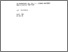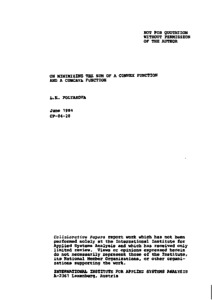# On Minimizing the Sum of a Convex Function and a Concave Function

Polyakova, L.N. (1984). On Minimizing the Sum of a Convex Function and a Concave Function. IIASA Collaborative Paper. IIASA, Laxenburg, Austria: CP-84-028Preview Text CP-84-028.pdf Download (196kB) | Preview

## Abstract

We consider here the problem of minimizing a particular subclass of quasidifferentiable functions: those which may be represented as the sum of a convex function and a concave function. It is shown that in an n-dimensional space this problem is equivalent to the problem of minimizing a concave function on a convex set. A successive approximations method is suggested; this makes use-of some of the principles of epsilon-steepest-descent-type approaches.

Item Type: Monograph (IIASA Collaborative Paper) quasidifferentiable functions, convex functions, concave functions, epsilon-steepest-descent methods System and Decision Sciences - Core (SDS) IIASA Import 15 Jan 2016 01:55 27 Aug 2021 17:12 https://pure.iiasa.ac.at/2549View Item